# Welcome to the Thermal Wizard

Use these quick calculators to understand common heat transfer problems and thermal phenomena. Thermal Wizard is developed and maintained by Maya HTT.

At Maya HTT, we know thermal simulation, on Earth and in space. Learn more about our software and services.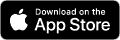# Our calculators: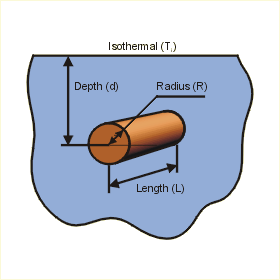This page calculates the temperature (Tc ) of an isothermal (constant temperature) cylinder embedded in a semi-infinite medium at a specified depth (d) from an isothermal surface. Define the cylinder's radius, length, depth, and the heat load, as well as the conductivity and the isothermal temperature applied to the medium surrounding the cylinder. This page will calculate the cylinder temperature. Do you want to know more ?

Embedded Cylinder and Flow Conditions

 Description Symbol Value Units Radius R Meters Length L Meters Depth d Meters Heat Load Q W

Conducting Medium's Properties (Default is for Epoxy [high fill], Units in SI)

 Description Symbol Value Units Conductivity k W/(m-oC) Isothermal Temperature Ti oC

Results for Isothermal Embedded Cylinder

 Description Symbol Value Units Conduction Shape Factor = sf None Embedded Cylinder Temperature = q/(k*sf)+Ti Tc oC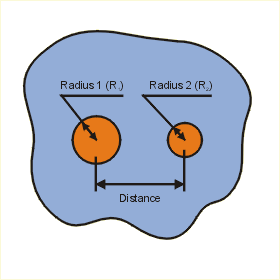This page calculates the temperature (T1) of one isothermal (constant temperature) cylinder based on conduction in an infinite medium to a second embedded cylinder at known temperature (T2). Heat (Q) is conducted from cylinder 1 to 2. Define the radii of the cylinders, the length (both cylinders are assumed to have the same length), the temperature of one cylinder, the distance between the cylinders and the heat flow. This page will calculate their corresponding shape factor and the temperature of the embedded cylinder. Do you want to know more ?

Embedded Cylinders and Flow Conditions

 Description Symbol Value Units Cylinder 1 Radius R1 Meters Cylinder 2 Radius R2 Meters Cylinder Length L Meters Cylinder 2 Temperature T2 oC Distance d Meters Heat Flow from Cylinder 1 to 2 Q W

Conducting Medium's Properties (Default is for Epoxy [high fill], Units in SI)

 Description Symbol Value Units Conductivity k W/(m-oC)

Results for two Isothermal Embedded Cylinders

 Description Symbol Value Units Conduction Shape Factor = 2*pi*L/cosh-1((d2-R12-R22)/(2*R1*R2)) sf None Embedded Cylinder 1 Temperature = q/(k*sf)+T2 T1 oCThis page calculates the isothermal (constant temperature) embedded rectangular volume temperature (Tb) and the shape factor (sf) of a rectangular volume embedded in a semi-infinite medium having isothermal surface. Define the rectangular volume's width, length, height, depth, and the heat flow, as well as the conductivity and the isothermal temperature applied to the medium surrounding the rectangular box. This page will calculate the rectangular box temperature and the corresponding shape factor. Do you want to know more ?

Embedded rectangular box and Flow Conditions

 Description Symbol Value Units Width W Meters Length L Meters Height H Meters Depth d Meters Heat Flow q W

Conducting Medium's Properties (Default is for Epoxy [high fill], Units in SI)

 Description Symbol Value Units Conductivity k W/(m-oC) Isothermal Temperature Ti oC

Results for Isothermal Embedded rectangular box

 Description Symbol Value Units Conduction Shape Factor = 1.685*L*(log(1+d/W))-0.59*(d/H)-0.078 sf None Embedded rectangular box Temperature = q/(k*sf)+Ti Tb oC

Material Properties

Material Conductivity (W/m-C) Density (kg/m)3 Specific Heat (J/kg-C)
Material Conductivity
W/m-C
Density
kg/m3
Specific Heat
J/kg-C

Properties at Atmospheric Pressure

Temperature
C
Conductivity
W/m-C
Density
kg/m3
Specific Heat
J/kg-C
Dynamic Viscosity
kg/m-s
Kinematic Viscosity
m2/s
Thermal Diffusivity
m2/s

Properties at Atmospheric Pressure

Gas Temperature
C
Conductivity
W/m-C
Density
kg/m3
Specific Heat
J/kg-C
Dynamic Viscosity
kg/m-s
Kinematic Viscosity
m2/s
Thermal Diffusivity
m2/s
Water (Saturated) - Thermal Properties
Temperature
C
Conductivity
W/m-C
Density
kg/m3
Specific Heat
J/kg-C
Dynamic Viscosity
kg/m-s
Liquid Temperature
C
Conductivity
W/m-C
Density
kg/m3
Specific Heat
J/kg-C
Kinematic Viscosity
m2/s
Thermal Diffusivity
m2/s
C.T.E.
1/C

# Material Property Libraries on the Web

Below is a list of links to other material property databases on the Web.

MatWeb - Free Material Database

IDES Plastic Materials Database - Prospector Products

Metal Suppliers On-Line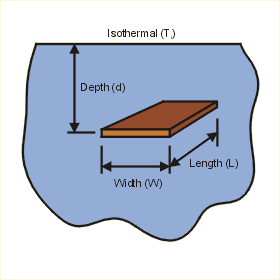This page calculates the plate temperature (Tp) for an isothermal (constant temperature) thin flat plate embedded in a semi-infinite medium with an applied isothermal temperature. Define the width, length, depth, and the heat load of the plate, as well as the conductivity and the isothermal temperature applied to the medium surrounding the plate. This page will calculate the plate temperature. Do you want to know more ?

Embedded Plate and Flow Conditions

 Description Symbol Value Units Width W Meters Length L Meters Depth d Meters Heat Load Q W

Conducting Medium's Properties (Default is for Epoxy [high fill], Units in SI)

 Description Symbol Value Units Conductivity k W/(m-oC) Isothermal Temperature Ti oC

Results for Isothermal Embedded Plate

 Description Symbol Value Units Conduction Shape Factor = sf None Embedded Plate Temperature = q/(k*sf)+Ti Tp oC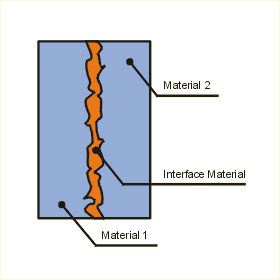This page calculates the temperature difference and the thermal contact conductance (thermal resistance) for an interface formed by two conforming, rectangular, rough surfaces as a function of contact pressure in the low pressure range. Define the surface properties and the interface material , as well as the conductivity and the isothermal temperature applied to the medium surrounding the plate. the interstitial material can be either a gas or a liquid (gas parameter is zero). This page will calculate the temperature difference and the thermal resistance between the two surfaces. Do you want to know more ?

Material and State Conditions (Choose a defined materials or enter your own data)

 Description Symbol Value Units Contact Pressure of Surfaces P MPa Width of Common Surface W m Length of Common Surface L m Heat Load on Surface 1 q W Surface 1 User Defined Al 5052 Al 6061 Al 6063-T5 Aluminum Nitride Alumina (96%Al2O3) Copper Microhardness of Surface 1 HC1 MPa Effective RMS Surface Roughness 1 sig1 micrometer Thermal Conductivity of Surface 1 k1 W/(m oC) Absolute Asperity Slope = 0.125 (sig1)0.402 m1 None Surface 2 User Defined Al 5052 Al 6061 Al 6063-T5 Aluminum Nitride Alumina (96%Al2O3) Copper Microhardness of Surface 2 HC2 MPa Effective RMS Surface Roughness 2 sig2 micrometer Thermal Conductivity of Surface 2 k2 W/(m oC) Absolute Asperity Slope = 0.125 (sig2)0.402 m2 None Interstitial Material User Defined Air Helium Thermal Grease Doped Thermal Grease Thermal Conductivity of Gap Substance k W/(m oC) Gas Parameter (@ T0 = 50oC and P0 = 1 atm) (M0 0 for liquid) M0 m Average Interface Thickness t m Gas Temperature (needed if substance is a gas) Tg oC Gas pressure (needed if substance is a gas) Pg kPa

Results for Thermal Grease Interface Resistance

 Description Symbol Value Units Microhardness used in Calculation HC MPa Effective RMS Surface Roughness = (sig12+sig22)1/2 sig micrometer Effective Mean Absolute Asperity Slope = 0.125 (sig)0.402 m None Harmonic Mean Thermal Conductivity = 2k1k2/(k1+k2) ks W/(m oC) Contact Conductive HTC1 = 1.25k1(m1/sig1)(P/H1)0.95 hc1 W/(m2 oC) Contact Conductive HTC2 = 1.25k2(m2/sig2)(P/H2)0.95 hc2 W/(m2 oC) Contact Conductive HTC = 1.25ks(m/sig)(P/HC)0.95 hc W/(m2 oC) Gas Parameter = M0(T/T0)(Pg,0/Pg) Mg m Effective Gap Thickness1 = 1.53sig1(P/H1)-0.097 Y1 m Effective Gap Thickness2 = 1.53sig2(P/H2)-0.097 Y2 m Effective Gap Thickness = 1.53sig(P/HC)-0.097 Y m Gap Conductive HTC1 = kg/ (Y1+ M) hg1 W/(m2 oC) Gap Conductive HTC2 = kg/ (Y2+ M) hg2 W/(m2 oC) Gap Conductive HTC = kg/ (Y+ M) hg W/(m2 oC) Joint Conductive HTC1 = hc1 + hg1 hj1 W/(m2 oC) Joint Conductive HTC2 = hc2 + hg2 hj2 W/(m2 oC) Joint Conductive HTC = hj W/(m2 oC) Interface Thermal Resistance = 1/ (W*L*hj) rj oC/W Temperature Difference = q/ (W*L*hj) T1-2 > oC NOTE: HTC stands for Heat Transfer Coefficient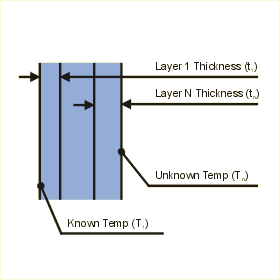This page calculates the temperature Tn at one side of an isothermal (constant temperature) multilayer wall. Define the height, depth, the low temperature on one side of the wall and a constant heat flow (from Tn to T1) through the wall. This page will calculate the higher temperature on the other side of the wall. The layers up to a zero value for Thickness or Conductivity will be included in the calculation. Do you want to know more ?

Multilayer Wall and Flow Conditions

 Description Symbol Value Units Height H Meters Depth D Meters Temperature 1 T1 oC Heat Flow q W
 Layer Sym Thickness (m) Sym Conductivity (W/m-oC) Layer 1 t1 k1 Layer 2 t2 k2 Layer 3 t3 k3 Layer 4 t4 k4 Layer 5 t5 k5 Layer 6 t6 k6 Layer 7 t7 k7 Layer 8 t8 k8 Layer 9 t9 k9 Layer 10 t10 k10

Results for Isothermal Multilayer Wall

 Description Symbol Value Units Temperature N =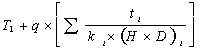Tn oC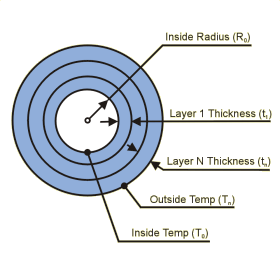This page calculates the temperature rise across a multilayer cylinder for a heat load (Q) applied to its inner surface at r0 and flowing to the outer surface. Define the cylinder's inside radius, length and the heat load conducted through the layers. This page will calculate the temperature rise between the inner and outer surfaces of this cylinder stack. For a positive heat load T0 > Tn and for a negative heat load Tn > T0. Do you want to know more ? Verification Test Case

Multilayer Cylinder and Flow Conditions

 Description Symbol Value Units Inner Radius R0 Meters Length L Meters Heat Flow from Inner to Outer Surface Q W

 Layer Thickness and Conductivity (First zero value ends the list) Sym Thickness (m) Sym Conductivity (W/m-oC) Layer 1 Thickness and Conductivity t1 k1 Layer 2 Thickness and Conductivity t2 k2 Layer 3 Thickness and Conductivity t3 k3 Layer 4 Thickness and Conductivity t4 k4 Layer 5 Thickness and Conductivity t5 k5 Layer 6 Thickness and Conductivity t6 k6 Layer 7 Thickness and Conductivity t7 k7 Layer 8 Thickness and Conductivity t8 k8 Layer 9 Thickness and Conductivity t9 k9 Layer 10 Thickness and Conductivity t10 k10

Results for Isothermal Multilayer Cylinder

 Description Symbol Value Units Outer Temperature =T oCThis page calculates the net radiant heat exchange for two isothermal (constant temperature) parallel flat black plates of equal size. Define the plate size, spacing, and the plate temperatures. This page will calculate the view factor between plates 1 and 2 and calculate the net radiant heat exchange between the two plates using this view factor. Do you want to know more ?

 Description Symbol Value Units Plate Length L Meters Plate Width W Meters Spacing (between plates) D Meters Plate 1 Temperature T1 K Plate 2 Temperature T2 K

Results for Isothermal Plates

 Description Symbol Value Units x = L/D x None y = W/D y None A = ln((1+x2)*(1+y2)/(1+x2+y2))0.5 A None B = y*(1+x2)0.5*tan-1(y/(1+x2)0.5) B None C = x*(1+y2)0.5*tan-1(x/(1+y2)0.5) C None D = y*tan-1(y)+x*tan-1(x) D None E = 2/(pi*x*y) E None View Factor(1-2) = (A+B+C-D)*E VF(1-2) None Heat Radiated = 56.69E-9*VF(1-2)*L*W*(T14-T24) q W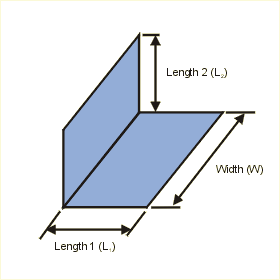This page calculates the net radiant heat exchange for two isothermal (constant temperature) perpendicular flat black plates of different lengths but equal widths. Define the plate size, spacing, and the plate temperatures. This page will calculate the view factor between plates 1 and 2 and calculate the net radiant heat exchange between the two plates using this view factor. Do you want to know more ?

 Description Symbol Value Units Plate 1 Length L1 Meters Plate 2 Length L2 Meters Width W Meters Plate 1 Temperature T1 K Plate 2 Temperature T2 K

Results for Isothermal Plates

 Description Symbol Value Units x = L2/W x None y = L1/W y None z = x2+y2 z None A = ln((1+x2)*(1+y2)/(1+z)) A None B = y2*(ln(y2*(1+z)/(z*(1+ y2)))) B None C = x2*(ln(x2*(1+z)/(z*(1+ x2)))) C None D = y*tan-1(1/y)+x*tan-1(1/x)-(z)0.5*tan-1(1/z0.5) D None View Factor(1-2) = (1/4*(A+B+C)+D)/(pi*y) VF(1-2) None Heat Radiated = 56.69e-9*VF(1-2)*L1*W*(T14-T24) q WThis page calculates the net radiant heat exchange for two isothermal (constant temperature) parallel square flat black plates of different edge lengths. Define the edge length of the plates, spacing, and the plate temperatures. This page will calculate the view factor between plates 1 and 2 and calculate the net radiant heat exchange between the two plates using this view factor. Do you want to know more ?

 Description Symbol Value Units Plate 1 Edge Length L1 Meters Plate 2 Edge Length L2 Meters Spacing (between plates) D Meters Plate 1 Temperature T1 K Plate 2 Temperature T2 K

Results for Isothermal Plates

 Description Symbol Value Units x = L1/D*(1+L2/L1) x None y = L1/D*(1-L2/L1) y None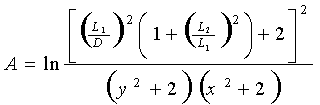A None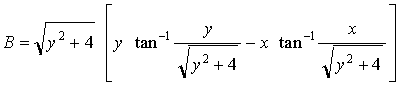B None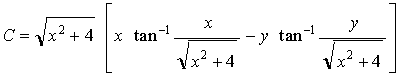C None View Factor(1-2) =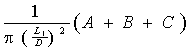VF(1-2) None Heat Radiated = 56.69e-9*VF(1-2)*L12*(T14-T24) q W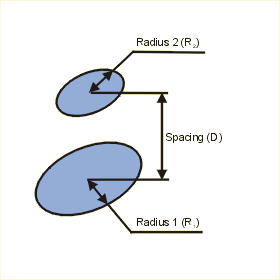This page calculates the net radiant heat exchange for two isothermal (constant temperature) parallel flat black disks. Define the disk radii, spacing, and the disk temperatures. This page will calculate the view factor between disks 1 and 2 and calculate the net radiant heat exchange between the two disks using this view factor. Do you want to know more ?

 Description Symbol Value Units Disk 1 Radius rad1 Meters Disk 2 Radius rad2 Meters Spacing (between disks) D Meters Disk 1 Temperature T1 K Disk 2 Temperature T2 K

Results for Isothermal Disks

 Description Symbol Value Units r1 = rad1/D r1 None r2 = rad2/D r2 None x = 1+(1+r22)/r12 x None View Factor(1-2) = 0.5*(x-(x2-4*(r2/r1)2)0.5) VF(1-2) None Heat Radiated = 56.69E-9*VF(1-2)*pi*rad12*(T14-T24) q W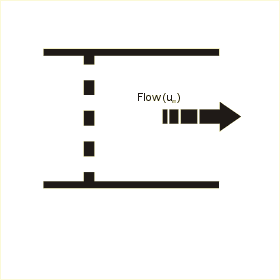This page calculates the head loss coefficient and head loss of a fluid across a screen (circular metal wire mesh) inside a pipe. The Reynolds number is assumed to be greater than 103. Define the free area ratio and the flow velocity in the pipe. The head loss across the screen will be calculated. Do you want to know more ?

Screen and Flow Properties

 Description Symbol Value Units Free Area Ratio (Open Area / Total Area) FAR None Mean Flow Velocity in the Pipe um m/s

Results for Head Loss of Screen

 Equations Used Symbol Value Units Head Loss Coefficient =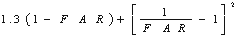kloss None Head Loss = kloss * (um2 / (2 * g) ) hL MetersThis page calculates the head loss coefficient and head loss of a fluid in across a thin perforated plate (plate thickness / hole hydraulic diameter < 0.015) inside a pipe. Reynolds number is assumed greater than 105. Define the free area ratio (open/total area) and the mean flow velocity in the pipe. The head loss across the perforated plate will be calculated. Do you want to know more ?

Perforated Plate and Flow Properties

 Description Symbol Value Units Free Area Ratio FAR None Flow Velocity in the Pipe um m/s

Results for Head Loss of Perforated Plate

 Equations Used Symbol Value Units Head Loss Coefficient =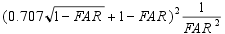kloss None Head Loss = kloss * (um2 / (2 * g) ) hL Meters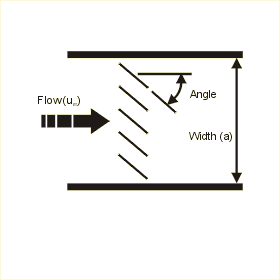This page calculates the head loss coefficient and head loss of a fluid across thin-walled parallel louvers inside a tube of square cross section. Define the number of louvers, angle and flow conditions in the tube. The head loss across the louvers will be calculated. Do you want to know more ?

Tube and Flow Properties

 Description Symbol Value Units Number of Louvers in Tube N 2 3 4 5 6 None Angle of Louvers in Tube A 0 10 20 30 40 50 60 70 80 Degrees Flow Velocity in the Tube um m/s Width of the Tube a Meters Kinematic Viscosity v kg/m-s

Results for Head Loss of Louvers

 Equations Used Symbol Value Units kqu = Function of Angle and Number of Louvers kqu None Hydraulic Diameter of the Tube = a Dh Meters Reynolds Number = um * Dh / v Re None X =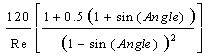X None Head Loss Coefficient = X + kloss None Head Loss = kloss * (um2 / (2 * g) ) hl Meters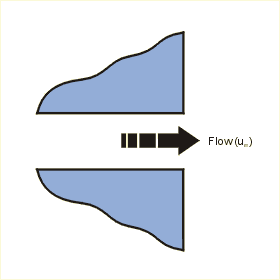This page calculates the head loss of a fluid at an abrupt pipe exit. Note that the head loss coefficient is 1.0 for an abrupt pipe exit. Define flow velocity in the pipe. The head loss at the pipe exit will be calculated. Do you want to know more ?

Pipe and Flow Properties

 Description Symbol Value Units Flow Velocity in the Pipe um m/s

Results for Head Loss of Pipe Entrance

 Equations Used Symbol Value Units Head Loss Coefficient kloss 1 None Head Loss = kloss * (um2 / (2 * g) ) hL Meters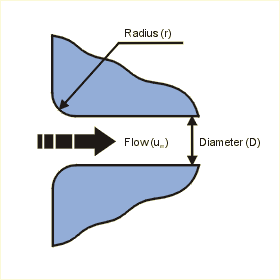This page calculates the head loss coefficient and head loss of a fluid at a smooth bellmouth pipe entrance. Define the radius of the bellmouth entrance and the hydraulic diameter of the pipe and enter the velocity of the fluid in the pipe. The headloss at the pipe entrance will be calculated. Do you want to know more ?

Pipe and Flow Properties

 Description Symbol Value Units Bellmouth Radius r Meters Hydraulic Diameter Dh Meters Flow Velocity in the Pipe um m/s

Results for Head Loss of Pipe Entrance

 Equations Used Symbol Value Units Diameter Ratio = r / Dh x None Head Loss Coefficient kloss None Head Loss = kloss * (um2 / (2 * g) ) hL Meters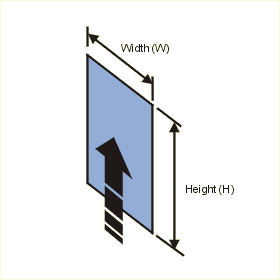This page calculates the convective and radiative heat transfer from an isothermal vertical plate. The average convective heat transfer coefficient is calculated assuming natural convection conditions. The convection calculation automatically switches between laminar and turbulent convection correlations based on Rayleigh number. The plate transfers heat from only one of its sides. It is assumed to be insulated on the other. Define the plate size, surface temperature and ambient temperature. You also need to define the fluid properties. This page will calculate a heat transfer coefficient based on the flow conditions as well as the heat transfer by convection and radiation from the isothermal plate. To account for convection only, make the emissivity equal to 0. Similarly, to account for radiation only, make the tehrmal conductivity = 0. Do you want to know more ?

Plate and Flow Conditions

Fluid Properties at Film Temperature, Except for Density. (Defaults are for Air at 20C)

 Description Symbol Value Units Film Temperature = (Tp+Ta)/2 Tf oC Specific Heat Cp J/kg-oC Coefficient of Thermal Expansion B 1/K Thermal Conductivity k W/m-oC Dynamic Viscosity µ kg/m-s Density at reference temperature of 20 C rhoref kg/m3 Density calculated at film temperature Tf rho kg/m3 Emissivity e None

Plate Dimensions

 Description Symbol Value Units Plate Width (across flow) W Meters Plate Height (with flow) H Meters Plate Temperature Tp oC Ambient Temperature Ta oC

Results for Plate

 Description Symbol Value Units Prandlt Number = v/a Pr None Grashof Number = g*cte*(Tp-Ta)*H3/v2 Gr None Rayleigh Number = Gr*Pr Ra None Nusselt= Nu None Average Heat Transfer Coefficient = Nu*k/H h W/m2-C Convective HeatTransfer = h*W*H*(Tp-Ta) qconv W Radiative Heat Transfer= Sigma*W*H*e*(Tp4-Ta4) qrad W Total Heat Transfer = qconv + qrad qtot W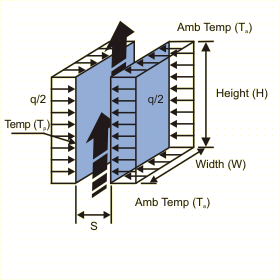This page calculates the average heat transfer coefficient and surface temperature for an isoflux (constant and uniform flux) parallel plate vertical channel open to ambient from both ends in a natural convection environment. The convection calculation are based on Rayleigh number. Define the channel size, uniform heat load and ambient air fluid temperature. You also need to define the fluid properties. This page will calculate a heat transfer coefficient based on the flow conditions as well as the temperature of the plates. Do you want to know more ?

Channel and Flow Conditions

Fluid Properties at Film Temperature (Default is for Air at 20C)

 Description Symbol Value Units Film Temperature = (Tp+Ta)/2 Tf oC Specific Heat Cp J/kg-oC Coefficient of Thermal Expansion B 1/K Thermal Conductivity k W/m-oC Dynamic Viscosity µ kg/m-s Density rho kg/m3

Channel Dimensions

 Description Symbol Value Units Channel Width (across flow) W Meters Channel Height (with flow) H Meters Channel Gap S Meters Total uniform heat load on plates q Watts Ambient Temperature Ta oC

Results for Plate

 Description Symbol Value Units Flux = q / (2*W*H) q" W/m2 Prandlt Number = Cp*µ / k Pr None Rayleigh Number = g*B*q"*S4*rho2*Cp / (µ*k2) Ra* None Ra*S/H Ra*S/H None Nusselt Number = ( 48*H /( Ra*S) + 2.51 / (Ra*S / H)(2/5) )-0.5 Nu None Average Heat Transfer Coefficient = Nu*k/S h W/m2-C Plates temperature Tp oC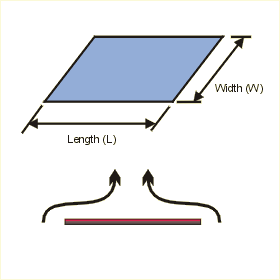This page calculates the average heat transfer coefficient and heat convected from an isothermal (constant temperature) heated horizontal flat plate's upper surface at a specified temperature in a natural convection environment. The convection calculation automatically switches between calculation methods based on Rayleigh number. Define the plate size, surface temperature, ambient air fluid temperature. You also need to define the fluid properties. This page will calculate a heat transfer coefficient based on the flow conditions as well as the heat transfer by convection from the isothermal plate. Do you want to know more ?

Plate and Flow Conditions

Fluid Properties at Film Temperature, Except for Density. (Defaults are for Air at 20C)

 Description Symbol Value Units Film Temperature = (Tp+Ta)/2 Tf oC Specific Heat Cp J/kg-oC Coefficient of Thermal Expansion B 1/K Thermal Conductivity k W/m-oC Dynamic Viscosity µ m2/s Density at reference temperature of 20 C rhoref kg/m3 Density calculated at film temperature Tf rho kg/m3 Emissivity e None

Plate Dimensions

 Description Symbol Value Units Plate Width W Meters Plate Length L Meters Plate Temperature Tp oC Ambient Temperature Ta oC

Results for Plate

 Description Symbol Value Units Prandtl Number = v/a Pr None Grashof Number = g*cte*(Tp-Ta)*((W*L)/(2W+2L)3)/v2 Gr None Rayleigh Number = Gr*Pr Ra None Nusselt Number = Nu None Average Heat Transfer Coefficient = Nu*k/((W*L)/(2W+2L)) h W/m2-C Convective Heat Transfer = h*W*L*(Tp-Ta) qconv W Radiative Heat Transfer = Sigma*W*L*e*(Tp4-Ta4) qrad W Total Heat Transfer = qconv + qrad qtot W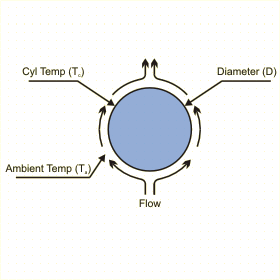This page calculates the heat transfer coefficient, heat convected and heat radiated from an isothermal horizontal cylinder assuming natural convection. The convection calculation is based on Rayleigh number and is valid for Rayleigh numbers between 10-5 and 1012. The ends of the cylinder are assumed to be adiabatic. Define the channel size, cylinder temperature and ambient air fluid temperature. You also need to define the fluid properties. This page will calculate a heat transfer coefficient based on the flow conditions as well as the heat convected from the cylinder. To account for convection only, make the emissivity equal to 0. Similarly, to account for radiation only, make the tehrmal conductivity = 0. Do you want to know more ?

Cylinder and Flow Conditions

Fluid Properties at Film Temperature (Default is for Air at 20C)

 Description Symbol Value Units Film Temperature = (Tc+Ta)/2 Tf oC Specific Heat Cp J/kg-oC Coefficient of Thermal Expansion B 1/K Dynamic Viscosity µ kg/m-s Density at reference temperature of 20 C rhoref kg/m3 Density calculated at film temperature Tf rho kg/m3 Thermal Conductivity k W/m-oC Emissivity e None

Cylinder Dimensions

 Description Symbol Value Units Length L Meters Diameter D Meters Cylinder Temperature Tc oC Ambient Temperature Ta oC

Results for Cylinder

 Description Symbol Value Units Prandlt Number = Cp*µ / k Pr None Area = PI * D A m2 Rayleigh Number = gB rho2Cp(Tc-Ta)D3 / kµ Ra None Nusselt = {0.60+(0.387RaD1/6) / [1+(0.559/Pr)9/16](8/27)}2 Nu None Average Heat Transfer Coefficient = Nu*k/HD h W/m2-C Convective Heat Transfer = hA(Tc-Ta) qconv W Radiative Heat Transfer qrad W Total Heat Transfer q W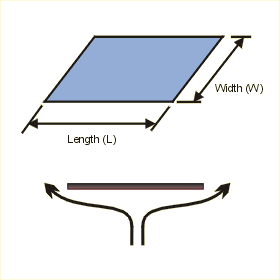This page calculates the average heat transfer coefficient and heat convected from an isothermal (constant temperature) heated horizontal flat plate's lower surface at a specified temperature in a natural convection environment. Define the plate size, surface temperature, ambient air fluid temperature. You also need to define the fluid properties. This page will calculate a heat transfer coefficient based on the flow conditions as well as the heat transfer by convection from the isothermal plate. Do you want to know more ?

Plate and Flow Conditions

Fluid Properties at Film Temperature, Except for Density. (Defaults are for Air at 20C)

 Description Symbol Value Units Film Temperature = (Tp+Ta)/2 Tf oC Specific Heat Cp J/kg-oC Coefficient of Thermal Expansion B 1/K Thermal Conductivity k W/m-oC Dynamic Viscosity µ kg/m-s Density at reference temperature of 20 C rhoref kg/m3 Density calculated at film temperature Tf rho kg/m3 Emissivity e None

Plate Dimensions

 Description Symbol Value Units Plate Width (across flow) W Meters Plate Length (along flow) L Meters Plate Temperature Tp oC Ambient Temperature Ta oC

Results for Plate

 Description Symbol Value Units Prandtl Number = v/a Pr None Grashof Number = g*cte*(Tp-Ta)*((W*L)/(2W+2L))3/v2 Gr None Rayleigh Number = Gr*Pr Ra None Nusselt Number = 0.27(Gr*Pr)0.25 Nu None Average Heat Transfer Coefficient = Nu*k/((W*L)/(2W+2L)) h W/m2-C Convective Heat Transfer = h*W*L*(Tp-Ta) qconv W Radiative Heat Transfer = Sigma*W*L*e*(Tp4-Ta4) qrad W Total Heat Transfer = qconv + qrad qtot W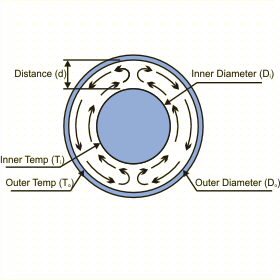This page calculates the natural convection heat transfer between two concentric cylinders maintained at constant temperatures. The calculation is based on Rayleigh number and is valid for Rayleigh numbers below 107.  Define the dimensions of the cylinders and their temperatures. You also need to define the fluid properties. This page will calculate the heat convected between the cylinders based on the flow conditions. Do you want to know more ?

Cylinders and Flow Conditions

Fluid Properties at Film Temperature (Default is for Air at 20C)

 Description Symbol Value Units Film Temperature = (Ti+To)/2 Tf oC Specific Heat Cp J/kg-oC Coefficient of Thermal Expansion B 1/K Thermal Conductivity k W/m-oC Dynamic Viscosity µ kg/m-s Density at reference temperature of 20 C rhoref kg/m3 Density calculated at film temperature Tf rho kg/m3

Cylinders Dimensions

 Description Symbol Value Units Length L Meters Inner Cylinder Diameter Di Meters Outer Cylinder Diameter Do Meters Inner Cylinder Temperature Ti oC Outer Cylinder Temperature To oC

Results for Concentric Cylinders

 Description Symbol Value Units Gap between cylinders d m Effective conductivity keff W/m-oC Prandlt Number = Cp*µ / k Pr None Rayleighd = gB rho2Cp(Ti-To)d3 / kµ Rad None Rayleighc* = Rad (ln(Do/Di))4) / d3(Di-3/5+Do-3/5)5 Rac* None Heat transfer = 2Pi keff L (Ti-To) / ln(Do/Di) q W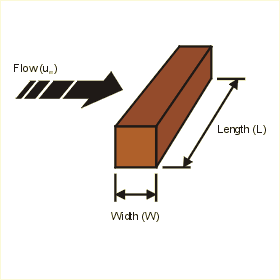This page calculates the average heat transfer coefficient and heat convected from an isothermal (constant temperature) square rod at a specified temperature in free stream flow. Define the rod size, surface temperature, ambient air fluid temperature and approach flow speed. You also need to define the fluid properties. This page will calculate a heat transfer coefficient based on the flow conditions as well as the heat transfer by convection from the isothermal square rod. Do you want to know more ?

Rod and Flow Conditions

 Description Symbol Value Units Rod Width W Meters Rod Length (perpendicular to flow) L Meters Rod Temperature Tr oC Ambient Temperature Ta oC Flow Velocity um m/s

Fluid Properties at Film Temperature (Default is for Air at 20C)

 Description Symbol Value Units Film Temperature = (Tr+Ta)/2 Tf oC Conductivity k W/m-oC Kinematic Viscosity v m2/s Thermal Diffusivity a m2/s

Results for Isothermal Rod

 Description Symbol Value Units Prandtl Number = v/a Pr None Reynolds Number = um*W/v Re None Nusselt Number = 0.102Re0.675 * Pr1/3 Nu None Heat Transfer Coefficient = Nu*k/W h W/m2-C Heat Dissipated from Rod = h*W*L*4*(Tr-Ta) q W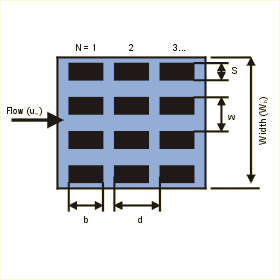This page calculates the heat transfer coefficient and temperature of a PCB board with components. Components are assume to be isothermal blocks. Temperatures at each row of components is calculated for the specified conditions. Define the PC board properties, component properties, and air flow properties. This page will calculate a heat transfer coefficient based on the flow conditions, calculate the resistance between the components and the air, as well as the component temperature. Do you want to know more ?

PC Board Properties

 Description Symbol Value Units Width of PCB (Lateral to Flow) Wb Meters Height of flow channel above PCB HF Meters Are their card guides at leading edges of PCB ? G Yes No None Conductivity of the board (no copper) kb W/m-oC Thickness of the board t Meters Volume of copper per area of PCB (Lateral to Flow) phi1 m3/m2 Volume of copper per area of PCB (Parallel to Flow) phi2 m3/m2 Conductivity of the PCB copper kc W/m-oC

Component Properties

 Description Symbol Value Units Number of components (Parallel to Flow) N 1 2 3 4 5 6 7 8 9 10 None Length (Parallel to Flow) b Meters Pitch (Parallel to Flow) d Meters Number of components (Lateral to Flow) M None Width (Lateral to Flow) S Meters Pitch (Lateral to Flow) w Meters Height (Protruding into Flow) e Meters Heat Load per Component q W Air gap between PCB and component del Meters Number of leads per component n None Length of leads L Meters Cross-sectional area of one lead Al m2 Thermal conductivity of leads kl W/m-oC

Air Flow Properties

 Description Symbol Value Units Ambient temperature (at entrance) Ta oC Average flow velocity in free flow area between boards um m/s Thermal conductivity of air ka W/m-oC Specific heat of air Cp J/kg-oC Air density rho kg/m3

Results for PC Board

 Equations Used Symbol Value Units Value of G for heat transfer coefficient calculation G None Flow area per PCB = Wb * HF - (M * S * e) a m2 Flow rate = um * a F m3/s Lateral conductance of the board = (kb * t) + (kc * phi1) C1 W/oC Streamwise conductance of the board = (kb * t) + (kc * phi2) C2 W/oC Resistance across air gap = del / (ka * S * b) rc oC/W Resistance across the leads = L / (kl * n * Al) rl oC/W Interface resistance = 1 / ( (1/rc) + (1/rl) ) Ri oC/W Exposed surface area = S * b + 2e (S + b) A1 m2
 Equations Used Symbol Units Heat transfer coefficient =h W/m2-oC Effective area =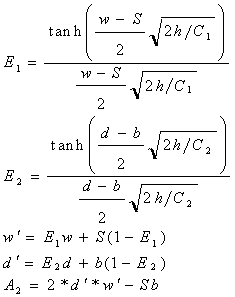A2 m2 Overall thermal resistance= 1 / (h x A1 + 1 / ( Ri + 1/ (A2 x h) ) ) R oC/W Local air temperature = (q*M*(N-1)) / (F*rho*Cp) Tl oC Component temperature = Tl + q * R Tc oC
 N h Units A2 Units R Units Tc Units 1 W/m2-oC m2 oC/W oC 2 W/m2-oC m2 oC/W oC 3 W/m2-oC m2 oC/W oC 4 W/m2-oC m2 oC/W oC 5 W/m2-oC m2 oC/W oC 6 W/m2-oC m2 oC/W oC 7 W/m2-oC m2 oC/W oC 8 W/m2-oC m2 oC/W oC 9 W/m2-oC m2 oC/W oC 10 W/m2-oC m2 oC/W oC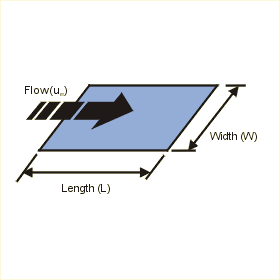This page calculates the average heat transfer coefficient and heat convected from an isothermal (constant temperature) flat plate at a specified temperature in free stream flow. The convection calculation automatically switches between laminar and turbulent convection correlations based on Reynolds number. Define the plate size, surface temperature, ambient air fluid temperature and approach flow speed. You also need to define the fluid properties. This page will calculate a heat transfer coefficient based on the flow conditions as well as the heat transfer by convection from the isothermal plate. Do you want to know more ?

Plate and Flow Conditions

Fluid Properties at Film Temperature (Default is for Air at 20C)

 Description Symbol Value Units Film Temperature = (Tp+Ta)/2 Tf oC Specific Heat Cp J/kg-oC Thermal Conductivity k W/m-oC Dynamic Viscosity µ kg/m-s Density rho kg/m3

Plate Dimensions

 Description Symbol Value Units Plate Width (across flow) W Meters Plate Length (along flow) L Meters Plate Temperature Tp oC Ambient Temperature Ta oC Flow Velocity um m/s

Results for Isothermal Plate

 Description Symbol Value Units Prandtl Number = v/a Pr None Reynolds Number = um*L/v Re None Nusselt Number = Nu None Heat Transfer Coefficient = Nu*k/L h W/m2-C Heat Dissipated from Plate = h*W*L*(Tp-Ta) q W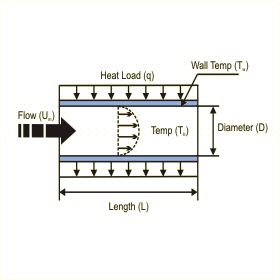This page calculates the heat transfer coefficient for fully developed flow in a smooth circular tube. The convection calculation automatically switches between laminar and turbulent convection correlations based on Reynolds number.  Define the tube size, heat load on the wall, fluid bulk temperature and flow speed. You also need to define the fluid properties. This page will calculate a heat transfer coefficient based on the flow conditions as well as the average tube wall temperature. Do you want to know more ?

Fluid Properties at Film Temperature (Default is for Air at 20C)

 Description Symbol Value Units Specific Heat Cp J/kg-oC Thermal Conductivity k W/m-oC Dynamic Viscosity µ kg/m-s Density Rho kg/m3

Tube Dimensions

 Description Symbol Value Units Tube Diameter D Meters Tube Length L Meters Heat Load q watts Bulk Fluid Temperature Tb oC Flow Velocity um m/s

Results for Circular Tube

 Description Symbol Value Units Prandtl Number = Cp*µ / k Pr None Reynolds Number = Rho*um*D / µ Re None Friction = f None Nusselt = Nu None Heat Transfer Coefficient = Nu*k / D h W/m2-C Area = Pi * D * L A m2 Average Wall Temperature = Tb + q / (h*A) Tw oCThis page calculates the mean heat transfer coefficient for simultaneously developing velocity and temperature fields in a circular smooth tube. The convection calculation automatically switches between laminar and turbulent convection correlations based on Reynolds number.  Define the tube size, heat load on the wall, fluid bulk temperature and flow speed. You also need to define the fluid properties. This page will calculate a heat transfer coefficient based on the flow conditions as well as the average tube wall temperature. Do you want to know more ?

Fluid Properties at Film Temperature (Default is for Air at 20C)

 Description Symbol Value Units Specific Heat Cp J/kg-oC Thermal Conductivity k W/m-oC Dynamic Viscosity µ kg/m-s Density Rho kg/m3

Tube Dimensions

 Description Symbol Value Units Tube Diameter D Meters Tube Length L Meters Heat Load q watts Bulk Fluid Temperature Tb oC Flow Velocity um m/s

Results for Circular Tube

 Description Symbol Value Units Prandtl Number = Cp*µ / k Pr None Reynolds Number = Rho*um*D / µ Re None Frictionf-dev = f None Nusseltf-dev = Nuf-dev None Nusseltm = Num None Heat Transfer Coefficient Multiplier = Num / Nuf-dev hmult None Mean Heat Transfer Coefficient = Num*k / D hm W/m2-C Area = Pi * D * L A m2 Average Wall Temperature = Tb + q / (hm*A) Tw oC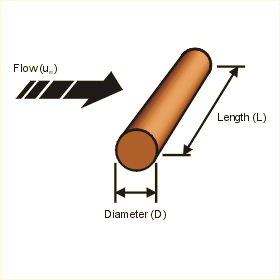This page calculates the average heat transfer coefficient and heat convected from an isothermal (constant temperature) circular rod at a specified temperature in free stream flow. Define the rod size, surface temperature, ambient air fluid temperature and approach flow speed. You also need to define the fluid properties. This page will calculate a heat transfer coefficient based on the flow conditions as well as the heat transfer by convection from the isothermal circular rod. Do you want to know more ?

Rod and Flow Conditions

 Description Symbol Value Units Rod Diameter D Meters Rod Length (perpendicular to flow) L Meters Rod Temperature Tr oC Ambient Temperature Ta oC Flow Velocity um m/s

Fluid Properties at Film Temperature (Default is for Air at 20C)

 Description Symbol Value Units Film Temperature = (Tr+Ta)/2 Tf oC Conductivity k W/m-oC Kinematic Viscosity v m2/s Thermal Diffusivity a m2/s

Results for Isothermal Rod

 Description Symbol Value Units Prandtl Number = v/a Pr None Reynolds Number = um*D/v Re None Nusselt Number = Nu None Heat Transfer Coefficient = Nu*k/D h W/m2-C Heat Dissipated from Rod = h*pi*D*L*(Tr-Ta) q W